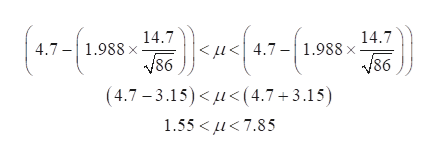# Based on interviews with86SARS​ patients, researchers found that the mean incubation period was 4.7​days, with a standard deviation of 14.7days. Based on this​ information, construct a​ 95% confidence interval for the mean incubation period of the SARS virus. Interpret the interval.The lower bound isnothingdays.​ (Round to two decimal places as​ needed.)

Question
6 views
Based on interviews with
86
SARS​ patients, researchers found that the mean incubation period was 4.7​days, with a standard deviation of 14.7
days. Based on this​ information, construct a​ 95% confidence interval for the mean incubation period of the SARS virus. Interpret the interval.
The lower bound is
nothing
days.​ (Round to two decimal places as​ needed.)
check_circle

Step 1

Confidence interval when population standard deviation is not known:

The formula of confidence interval for the population mean when population standard deviation is not given is as follows.

Step 2

Computing the 95% confidence interval for population mean:

Here, the sample mean (x-bar) is 4.7 days.

The sample standard deviation (s) is 14.7 and the sample size, n  is 86.

The confidence level is 0.95.

Thus, the level of significance, α is 0.05 (= 1 – 0.95).

The degrees of freedom (n − 1) is 85 (=86 − 1).

For 95% confidence interval, the critical value (t) is obtained as 1.988 using the EXCEL formula, (=T.INV.2T(0.05,85)).

Step 3

By substituting the values in the confidence interval formula, the 95% confidence i...help_outlineImage Transcriptionclose14.7 14.7 < fu<4.7-1.988 x 86 4.7-1.988 V86 (4.7-3.15)< u(4.7 3.15) 1.55 < μ< 7.85 fullscreen

### Want to see the full answer?

See Solution

#### Want to see this answer and more?

Solutions are written by subject experts who are available 24/7. Questions are typically answered within 1 hour.*

See Solution
*Response times may vary by subject and question.
Tagged in

### Hypothesis Testing